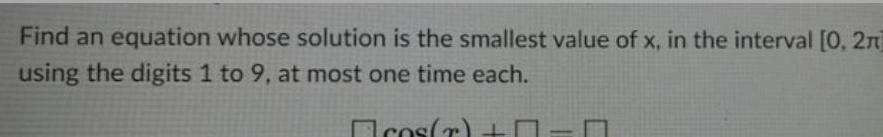Question:

# Find an equation whose solution is the smallest value of x,

Last updated: 8/12/2022Find an equation whose solution is the smallest value of x, in the interval [0, 2n] using the digits 1 to 9, at most one time each. Ecos(r) +